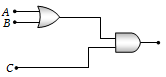NEET Physics Semiconductor Electronics Questions Solved

To get an output 1 from the circuit shown in the figure, the input must be(a) A = 0, B = 1, C = 0
(b) A = 1, B = 0, C = 0
(c) A = 1, B = 0, C = 1
(d) A = 1, B = 1, C = 0

Explanation is a part of a Paid Course. To view Explanation Please buy the course.

Difficulty Level: